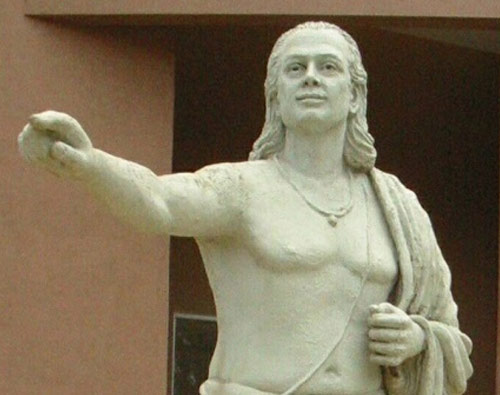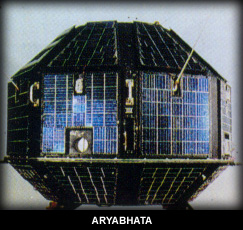A# DarlingFig 1. Statue depicting Aryabhata.Fig 2. India's first satellite.

Aryabhata was the first of the great astronomer-mathematicians from the classical age of Indian mathematics, which ran from about the 5th century AD to the 11th century. He was born in 476 AD, probably in Kusumapura, or present-day Patna, in eastern India and died in 550. He's sometimes referred as Aryabhata the Elder or Aryabhata the First because there was another Indian mathematician of the same name who lived later, in the 10th century.

Aryabhata wrote several treatises on mathematics and astronomy, only one of which, his great masterpiece, the Aryabhatiya, has survived to the present day. Written in verse, it contains one of the earliest treatments of algebra and some remarkably advanced astronomical knowledge. The mathematical part of the Aryabhatiya covers arithmetic, algebra, plane and spherical trigonometry, continued fractions, quadratic equations, sums-of-power series, and a table of sines.

It gives an approximation to pi with this description: "Add four to 100, multiply by eight, and then add 62,000. By this rule the circumference of a circle with a diameter of 20,000 can be approached."

This gives a value of 3.1416, which is accurate three decimal places. It's also been suggested that Aryabhata's use of the word "approached" indicates that he was aware of the fact that pi is incommensurable or irrational. If that's the case, it was a remarkable insight because the irrationality of pi wasn't actually proven until the 18th century.

The Aryabhatiya makes use of a place-value system for arithmetic, gives a formula for the area of a triangle, and deals with the concept of sine, which he calls ardha-jya or "half-chord". In fact, our word sine has come about, through a series of translations and mistranslations, of Arybhata's original name for the concept.

Since ancient times, Indian mathematicians had been fascinated by the problem of finding integer solutions to Diophantine equations – equations of the form ax + by = c, where a, b and c are all integers. In his commentary on the Aryabhatiya, the 7th century Indian mathematician Bhaskara, gives this example of such a problem:

Find the number which gives 5 as the remainder when divided by 8, 4 as the remainder when divided by 9, and 1 as the remainder when divided by 7.

This means finding a value N, such that N = 8x + 5 = 9y + 4 = 7z + 1. It turns out that the smallest value for N is 85. In general, Diophantine problems like this are notoriously difficult. Aryabhata's method of solving such problems is called the kuttaka method. Kuttaka means "pulverizing" or "breaking into small pieces", and the method involves a recursive algorithm for reducing the original factors to smaller numbers. It became the standard method for solving first-order Diophantine equations in Indian mathematics, and to begin with the whole subject of algebra was called kuṭṭaka-gaṇita or simply kuṭṭaka.

The Aryabhatiyaa contains some incredibly modern ideas in astronomy, far ahead of their time. It argues that the planets move in elliptical paths, that the apparent rotation of objects in the night sky is due to Earth's axial rotation, and that the Moon and planets shine by reflected sunlight. Aryabhata also correctly explained the causes of solar and lunar eclipses – improving upon the then-popular Indian belief that eclipses were the work of a demon called Rahu – and gave a value for the length of the year that's just a few hours longer than the one accepted today.

Finally, the Aryabhatiya is remarkable for its description of the relativity of motion. Aryabhata expressed the relativity of motion in this way: "Just as a man in a boat moving forward sees the stationary objects on the shore as moving backward, just so are the stationary stars seen by the people on earth as moving exactly towards the west."

Aryabhata's influence on Indian mathematics was profound. And through Arabic and other translations, his work in mathematics and astronomy was important in the development of these subjects, centuries later, in the West. India's first satellite Aryabhata and the lunar crater Aryabhata are both named in his honor.

## Indian's first satellite

Aryabhata was India's first satellite. The Soviet Union provided the launch vehicle and assisted India in developing Aryabhata, which carried out satellite technology tests and made observations of the upper atmosphere.

 launch date Apr 19, 1975 launch vehicle Cosmos-3M launch site Kapustin Yar orbit 398 × 409 km × 50.7° mass 360 kg